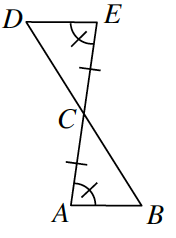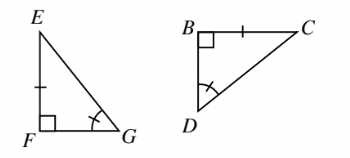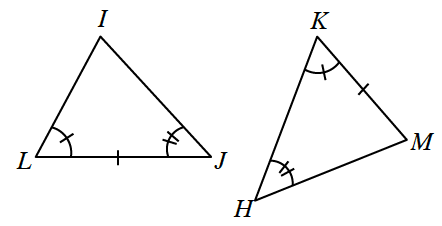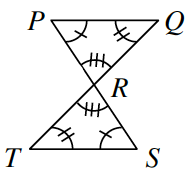### Home > INT1 > Chapter 7 > Lesson 7.1.5 > Problem7-59

7-59.

For each pair of triangles below, determine if the triangles are congruent. If the triangles are congruent, make a flowchart that justifies your conclusion. Make sure your flowchart states the triangle congruence condition that justifies your conclusion. If you cannot conclude that the triangles are congruent, explain why not.

Review Lesson 7.1.3 and Lesson 7.1.4.
Read the Math Notes box in lesson 7.1.7 on Triangle Congruence Conditions.

1. $ΔCAB ≅ Δ\text{ _____}$$ΔCED$; vertical angles are equal, $\text{ASA} ≅$.

1. $ΔCBD ≅ Δ\text{ _____}$$ΔEFG$; $\text{AAS} ≅$

1. $ΔLJI ≅ Δ\text{ _____}$1. $ΔPRQ ≅ Δ\text{ _____}$